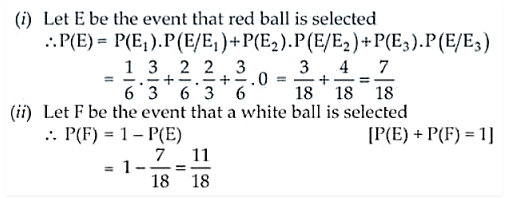# Three bags contain a number of red and white balls as follows:

Question:

Three bags contain a number of red and white balls as follows:

Bag 1 : 3 red balls, Bag 2 : 2 red balls and 1 white ball

Bag 3 : 3 white balls.

The probability that bag will be chosen and a ball is selected from it is i/6, i = 1, 2, 3. What is the probability that

(i) a red ball will be selected?

(ii) a white ball is selected?

Solution:

Given:

Bag 1 : 3 red balls,

Bag 2 : 2 red balls and 1 white ball

Bag 3 : 3 white balls

Now, let E1, E2 and E3 be the events of choosing Bag 1, Bag 2 and Bag 3 respectively and a ball is drawn from it.

And, we have

P(E1) = 1/6, P(E2) = 2/6 and P(E3) = 3/6Therefore, the required probabilities are 7/18 and 11/18.